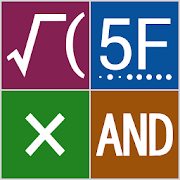Fantastic CalculatorEveryone
2
·
Offers in-app purchases
Feature
* The expression input box and the calculation result box are separated.
* Whenever expression changes, the result is displayed in real time.
* Histories of the calculation are memorized and an expression in the past can be recalled and used.
* Calculation of time (sexagesimal number) can be performed.
* It is possible to calculate hexadecimal numbers also, and they are displayed with bit pattern (binary).
* Modulo and power operation are possible in addition to the four arithmetic operations.
* Both "÷" and "/" can be used as the division symbol.
* Logical operations of each bit are possible and it is convenient for programmers.
* Many mathematical functions can be used and it can be calculated with parentheses. (Multiplication symbol can be omitted.)
* Trigonometric functions in radians and degrees are separate. Therefore, those mixed operation is possible.
* Numerical memories can be used.
* User definition functions can be used.
* Calculation results can be copied to the clipboard.

Functions Equipped
* Square root
* Cubic root
* Common logarithm
* Natural logarithm
* Reciprocal
* Absolute value
* Returns the fractional part of the parameter
* Hyperbolic functions
* Trigonometric functions (Angle expressed in radians)
* Inverse trigonometric functions (Angle expressed in radians)
* Trigonometric functions (Angle expressed in degrees)
* Inverse trigonometric functions (Angle expressed in degrees)
* Returns the integer rounded to the nearest
* Removes the fractional part of the parameter (Same as floor function in positive range and same as ceil function in negative range)
* Returns the integer rounded down (floor function)
* Returns the integer rounded up (ceil function)
* Factorial
* Bit inversion (with Integer conversion)

Attention
* Integer arithmetic is performed in 64-bit, and floating-point arithmetic is performed in significant digits 52 bits.
* However, calculation results are considered as follows.
434328865^2 - 434328864^2 = 868657729
68^13 MOD 77 = 19
* But it is safer to transform such a dangerous formula which is likely to carry out overflow.
(434328865 - 434328864) (434328865 + 434328864) = 868657729
(68^7 MOD 77) (68^6 MOD 77) MOD 77 = 19
* The real number (floating-point arithmetic) handled by a computer cannot avoid a rounding error. We consider it so that an error may not come out as much as possible. But keep in mind that it may shift slightly even when it should be a fitting answer.

Help
* Usage is written on help.
* Please refer to the following operation to display the help.
1. Press the ** key to switch the function indication.
2. Press the [?] key.

* You can double memories, user defined functions and histories. (a charged option)
The window for purchase appears when you tap Mf key twice.
Collapse

Review Policy
3.0
2 total
5
4
3
2
1

What's New

Version 1.1
* App name changed to "Fantastic Calculator"
* Both "÷" and "/" available for division symbol (possible to alternate by long tap)
* Improved help screen to proceed the option purchase

Version 1.0.3
* Bug fix about the abnormal termination on some models
Collapse

Updated
October 4, 2017
Size
2.4M
Installs
500+
Current Version
1.1
Requires Android
4.0.3 and up
Content Rating
Everyone
Interactive Elements
Digital Purchases
In-app Products
\$0.99 per item
Permissions
Offered By
AsaTech
Developer
Unumakawasaki-cho, Kakamigahara City, Gifu Prefecture, Japan